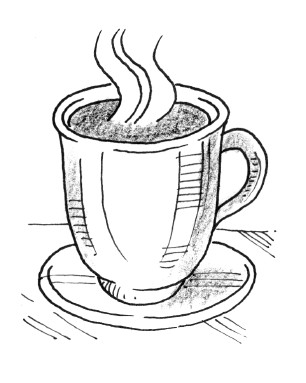### Home > CALC > Chapter 2 > Lesson 2.2.2 > Problem2-65

2-65.

A mug of hot coffee is poured and then set on the counter.

1. Sketch a feasible graph showing the temperature of the coffee as a function of time. Do not worry about units, just show the general behavior of the graph.

As the coffee cools, temperature will drop...
But will it approach a constant temperature (a horizontal asymptote)?
Or will it drop without bound (such that as $x → ∞$, $°F → − ∞$)?

2. Evaluate the following limit and translate the entire limit statement into a complete sentence.
$\lim\limits_ { \text{time} \rightarrow \infty } ( \text { temperature } ) =$

If you sketched a horizontal asymptote, then the limit should approach a constant value.
What does that horizontal asymptote represent? (use words)

If your sketch shows the temperature $→ −∞$, then the limit does not exist, but approaches $−∞$.
What does that represent? (use words)